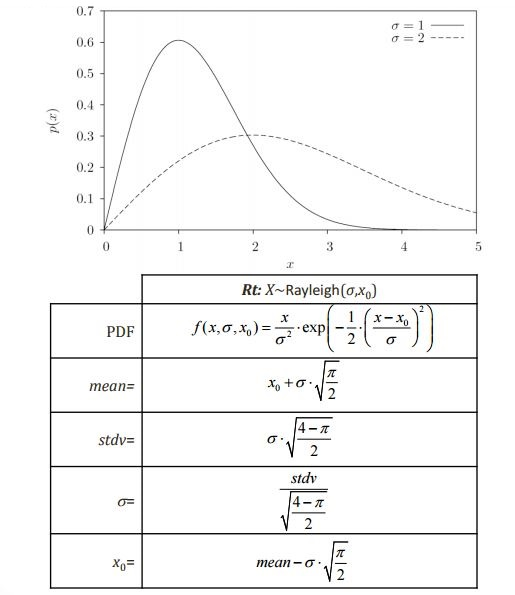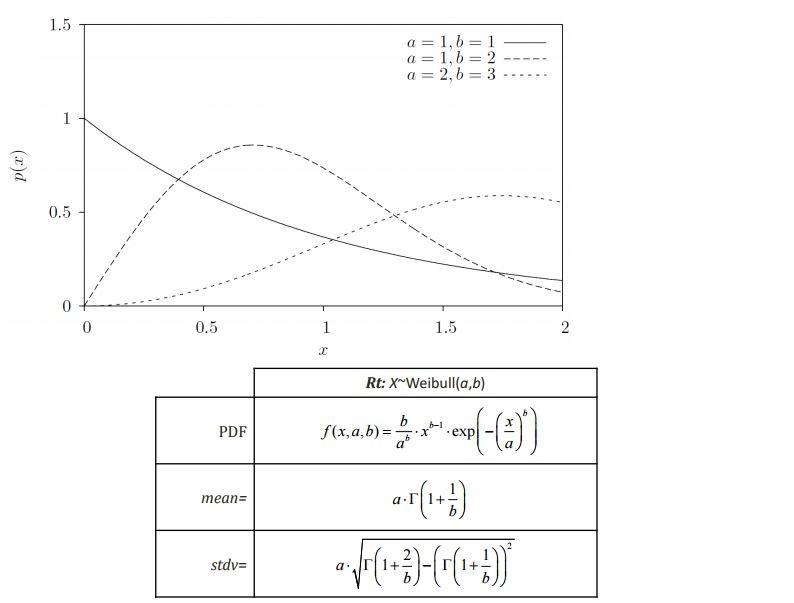﻿ Continuous Random Variable

# Continuous Random Variable

## Class Name

• RContinuousRandomVariable

## Location in Objects Pane

• Models > Parameter > Random Variable > Continuous

## Properties### Object Name

• Name of the object in Rt
• Allowable characters are upper-case and lower-case letters, numbers, and underscore (“_”).
• The name is unique and case-sensitive.

### Current Value

• Current realization of the random variable

### Distribution Type

• Probability distribution of the random variable
• The distribution type is followed by the distribution parameters inside parentheses.
• These parameters, respectively, correspond to Parameter 1 to Parameter 4 that appear as some other properties below.
• If, for instance, only two parameters appear inside parentheses for a particular distribution type, only Parameter 1 and Parameter 2 are applicable to that distribution.
• If the “User-defined” distribution is selected, two more properties are shown, namely, X Points and PDF Points, described below.

### Coefficient Of Variation

• Coefficient of variation of the random variable
• It is a standardized measure of dispersion of a probability distribution.
• If changed, the values of Standard Deviation and Parameter 1 to Parameter 4 properties are re-calculated.

### Uncertainty Type

• Indicates whether the random variable describes an “Aleatory” or an “Epistemic” uncertainty.
• This is employed, for instance, when computing model response standard deviation sensitivities in FORM.

### X Points

• Vector of random variable realizations for which the PDF values are given to the property below, i.e., PDF Points in a “User Defined” distribution.

### PDF Points

• Vector of PDF values of the random variable realizations that are given to the property above, i.e., X Points in a “User Defined” distribution.

### Alpha Importance Measure

• Element of the $$\boldsymbol{\alpha}$$ importance vector, computed in FORM, that corresponds to this random variable.
• $$\boldsymbol{\alpha}$$ is a unit vector that indicates the relative importance of the random variables involved in a FORM analysis when correlations are neglected/non-existent.

### Gamma Importance Measure

• Element of the $$\boldsymbol{\gamma}$$ importance vector, computed in FORM, that corresponds to this random variable.
• $$\boldsymbol{\gamma}$$ is a unit vector that indicates the relative importance of the random variables involved in a FORM analysis when correlations are considered.

### FOSM Importance Measure

• Element of the FOSM importance vector, $$\boldsymbol{\omega}$$, computed in FOSM, that corresponds to this random variable.
• $$\boldsymbol{\omega}$$ is a unit vector that indicates the relative importance of the random variables involved in a FOSM analysis.
• $$\boldsymbol{\omega}$$ is computed as
• $$\boldsymbol{\omega}\ = - {\nabla {g^T}{\mathbf{D _ X}} \over {\left\| {\nabla {g^T}{\mathbf{D_X}}} \right\|}}$$ where $$\nabla {g}$$ = gradient of the limit-state function, $$g$$, and $$\mathbf{D_X}$$ = standard deviation matrix of random variables, $$\mathbf{X}$$.

### Delta Sensitivity Measure

• Element of the $$\boldsymbol{\delta}$$ sensitivity vector, that corresponds to this random variable.
• $$\boldsymbol{\delta}$$ is a dimensionless vector that is computed by multiplying the standard deviation matrix by the gradient of the reliability index with respect to the mean of random variables.

### Eta Sensitivity Measure

• Element of the $$\boldsymbol{\eta}$$ sensitivity vector, that corresponds to this random variable
• $$\boldsymbol{\eta}$$ is a dimensionless vector that is computed by multiplying the standard deviation matrix by the gradient of the reliability index with respect to the standard deviation of random variables

### Kappa Sensitivity Measure

• Element of the $$\boldsymbol{\kappa}$$ sensitivity vector, that corresponds to this random variable.
• $$\boldsymbol{\kappa}$$ is a dimensionless vector equal to the gradient of the reliability index with respect to the coefficient of variation of random variables.

### Beta Sensitivity Wrt Mean

• Derivative of the reliability index, $${\beta}$$, with respect to the mean of this random variable

### Beta Sensitivity Wrt Standard Deviation

• Derivative of the reliability index, $${\beta}$$, with respect to the standard deviation of this random variable

### Remove

• Removes the object.

### Plot PDF

• Plots the probability density function of the random variable in the range of mean ± three standard deviations.

### Plot CDF

• Plots the cumulative distribution function of the random variable in the range of mean ± three standard deviations.

### Plot Inverse CDF

• Plots the inverse cumulative distribution function of the random variable in the range of mean ± three standard deviations

### Get PDF

• Prints the probability density function value for a given random variable realization on the output pane.

### Get CDF

• Prints the cumulative distribution function value for a given random variable realization on the output pane.

### Get Inverse CDF

• Prints the inverse cumulative distribution function value, i.e., the realization, for a given probability on the output pane.

## Types of Distribution

### Beta Distribution### Chi-Squared Distribution### Exponential Distribution### Gamma Distribution### Gumbel Distribution### Laplace Distribution### Logistic Distribution### Lognormal Distribution### Normal Distribution### Rayleigh Distribution### t Distribution### Uniform Distribution### Weibull Distribution### Wald Distribution### Levy Distribution### Irvin-Hall Distribution### Birnbaum-Saunders Distribution### Type-2 Gumbel Distribution### Pareto Distribution### F Distribution### Arcsine Distribution## References

• Der Kiureghian, A. (2005). First-and second-order reliability methods. In E. Nikolaidis, D. M. Ghiocel, & S. Singhal (Eds.), Engineering design reliability handbook. Boca Raton, Florida: CRC Press
• Der Kiureghian, A., & Ditlevsen, O. (2009). Aleatory or epistemic? Does it matter? Structural Safety, 31(2), 105–112